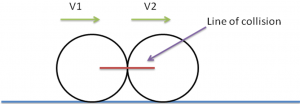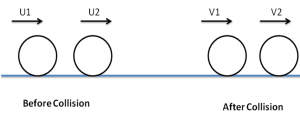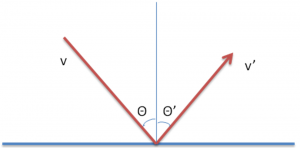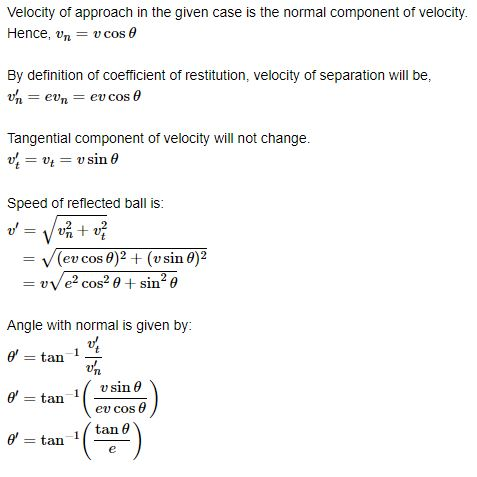# Collision- FAQ in JEE

Having a good knowledge of collisions is important in Physics. It is one of the vital topics from which questions are asked in JEE Exam every year. Some of the FAQ’s related to this topic is discussed below.

## Important Questions For Collision

1) What is a head-on collision and oblique collision?

Head-on Collision: It happens when velocities of both the particles are along the line of collision (as shown in the figure). This can be regarded as a collision in one dimension. A car accident is one of the basic examples of one dimension collision.Oblique Collision: It happens when any of the two bodies have a velocity at an angle with the line of collision. In case of an oblique collision, the component of velocity perpendicular to the line of the collision remains unchanged. This can be regarded as a collision in two dimensions.2) Explain the classification of collisions.

Collisions can be classified on the basis of whether kinetic energy remains constant or not. If the kinetic energy of the system remains constant then it is known as an elastic collision. While there are situations when some of the kinetic energy gets converted into heat, deformation of shape etc. These types of collisions are known as an inelastic collision.

We often define another quantity known coefficient of restitution (e). It is defined as the ratio of the velocity of separation by the velocity of approach along the line of collision. So,

Coefficient of restitution (e) = Relative Velocity after collision / Relative Velocity after collisionDepending on the values of e we can infer the type of collision.

When

e = 1, it is elastic collision

0 < e < 1, it is inelastic collision

e = 0, it is perfectly inelastic collision

3) A ball of mass m hits the floor with a speed v making an angle of incidence α with the normal. The coefficient of restitution is e. Find the speed of the reflected ball and the angle of reflection of the ball.Let the speed of the ball after the collision is v’ and the angle of reflection is ?’. The floor exerts a force on the ball along the normal and there is no force in the horizontal direction. Therefore the component of velocity along horizontal direction remains unchanged.4) What are some of the best books to refer for collisions in physics?

There are many good books on collision like D.C Pandey, H.C Verma, Halliday and Resnick. Apart from knowing concepts practice is very important for this topic.

To know more about different topics in physics join keep visiting BYJU’S.